Industry Article

# Resolving the Signal Part 11: Understanding How Power-Supply Noise Affects Delta-Sigma ADCs

December 11, 2018 by Bryan Lizon, Texas Instruments

## Part 11 of the Resolving the Signal series explores how power supplies contribute to unwanted noise, how to measure and quantify that noise, and how noise ends up impacting system performance.

Part 11 of the Resolving the Signal series explores how external power supplies for ADCs contribute to unwanted noise, how to measure and quantify that noise, and how noise ends up impacting system performance.

This article was co-authored by Ryan Andrews, applications engineer for precision analog-to-digital converters at Texas Instruments.

So far in “Resolving the Signal,” we’ve focused on noise contributed by analog components that could either be external to or integrated within your analog-to-digital converter (ADC). However, the final topic in this article series analyzes noise contributed by power supplies, which must originate outside of your device. Even though some ADCs might integrate power-management features, such as a low-dropout (LDO) regulator to clean up noisy supplies, or a charge pump to extend the input-voltage range, these features – and the ADC itself – still need power from an external source. And, like any other component in mixed-signal data-acquisition systems, power supplies contribute noise.

Fortunately, you can treat power-supply noise analysis similar to the other noise sources we’ve discussed in this article series. You can assume that your supplies will contribute some noise, although the impact on your system performance depends on the level and type of noise from the source. For example, a 3-V lithium-ion battery used in portable applications will generally be noisier and have more output-voltage variation than the precision bench-top power supply used to characterize an ADC. Once you’ve chosen the supply source and voltage rails for your application, there are methods you can take to reduce the impact that power-supply noise has on your ADC’s performance.

Since “Resolving the Signal” focuses on signal-chain design, let’s assume that your power supplies (and subsequent noise contributions) are fixed. In other words, we won’t discuss power-supply design techniques that could change the power-supply noise contribution. Instead, we’ll focus on how this noise affects your ADC’s output by discussing these concepts:

• Types and sources of power-supply noise.
• Measuring and quantifying power-supply noise rejection.
• How noise on each of the ADC’s supplies impacts system performance.

In part 12, we’ll use a precision ADC evaluation module (EVM) to apply the theory from part 11 to a real-world example, as well as discuss power-supply noise-mitigation techniques.

### Types and Sources of Power Supply Noise

For any analog signal chain, power ultimately comes from one of two sources: batteries or AC line voltage. Both power-source options are often followed by some form of power-supply conditioning circuitry that regulates power for the rest of the system. The supply source as well as the active and passive conditioning components will contribute some level of noise, which manifests itself in the power supply’s output as a variation from the expected voltage. This variation could appear as a steady DC shift in the output or as an AC signal at some frequency and amplitude riding on top of the output.

While the latter may seem specific to line-voltage powered systems, battery-powered systems may contain AC noise as well. The power-conditioning components themselves can contribute noise that impacts performance. These devices include LDOs, DC/DC converters, and switched-mode power supplies (SMPSs). LDOs primarily contribute thermal noise, like that associated with all electrical components. Switching devices add large current transients on top of thermal noise. Current transients generally consist of both a lower-frequency ripple (typically in the 100-kHz to 1-MHz range) as well as higher-frequency spikes (+100 MHz) centered on the nominal output voltage. Figure 1 shows an oscilloscope plot of switching ripple, transient spikes, and thermal noise.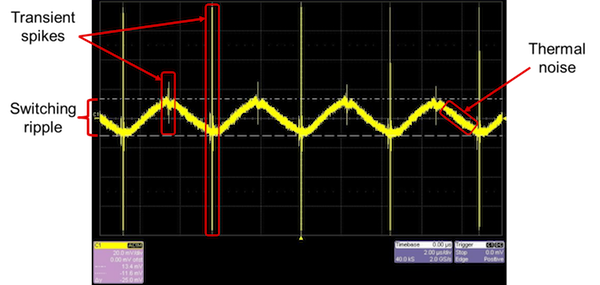##### Figure 1. SMPS noise (transients, ripple, and thermal noise)

In addition to power-supply conditioning components, AC noise can originate from other switching components that use the same supply – such as clocks and clock buffers – as well as from ambient lighting or other environmental elements.

Ultimately, your ADC power supplies will likely have some combination of both thermal and switching noise regardless of which power source you use. However, since power-supply noise is external to the ADC, semiconductor manufacturers can only specify how effective an ADC is at rejecting this noise. This specification is called power-supply rejection (PSR).

### Measuring and Quantifying Power Supply Noise Rejection

In ADCs, PSR describes the change in the ADC output (ΔVOUT) relative to changes in its power supply (ΔVSUPPLY). PSR is often given as a ratio expressed in decibels. This ratio is referred to as the power-supply rejection ratio (PSRR). Note that the absolute value is conventionally used to describe PSRR, since the negative sign is implied. Equation 1 calculates PSRR: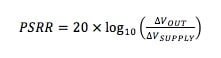You’ll find two different methods to specify PSRR in ADC data sheets: PSRRDC and PSRRAC. PSRRDC describes how much the ADC output changes due to a DC shift in its power supply, while PSRRAC describes how much power-supply noise appears in the output. Similar to the ADC noise-measurement discussion in part 2, the PSRR measurement method used by ADC manufacturers depends on the types of signals the ADC is intended to measure.

For example, low-speed sensor-measurement applications typically use ADCs optimized for DC performance, such as the Texas Instruments ADS1261, a very low-noise, 24-bit delta-sigma ADC that can sample up to 40 kilosamples per second (kSPS). Figure 2 shows the ADS1261’s data-sheet specification for PSRRDC since the signal passband is typically very narrow for this converter. The data sheet gives a minimum and typical PSRR specification for both the analog and digital power supplies.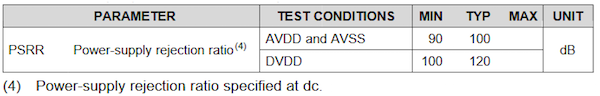##### Figure 2. ADS1261 PSRR for AVDD = 5 V, AVSS = 0 V, DVDD = 3.3 V, VREF = 2.5 V, gain = 1 V/V, output data rate (ODR) = 20 SPS

To measure PSRRDC, ADC manufacturers short the device’s inputs together, bias them to a common-mode voltage (VCM) typically near mid-supply, and measure the offset voltage at the ADC’s output. Then, the supply voltage is changed by 100 mV to see how much the offset voltage changes. Figure 3 shows a typical PSRRDC measurement setup, with the additional 100-mV offset voltage highlighted in red.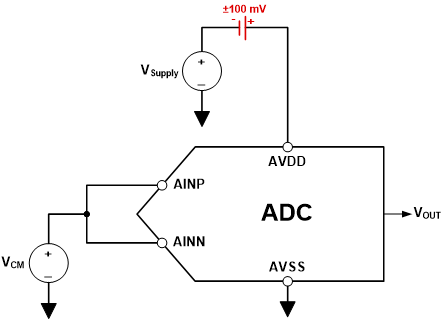##### Figure 3. PSRRDC measurement setup using ±100-mV offset on AVDD

As shown in Figure 2, the ADS1261’s typical PSRRDC is 100 dB for the analog supply, AVDD. You can input a PSRR of -100 dB and a ΔVSUPPLY of 100 mV into Equation 1 and solve for ΔVOUT to calculate the expected change in offset. From Equation 1, a 100-mV change in AVDD should produce a 1-µV change in offset voltage at the ADC output.

Figure 4 illustrates the output offset voltage’s variation due to the change in power supply in the time domain. The output offset voltage swing is centered on the ADS1261’s nominal offset voltage of 50 µV, which is specified using the same conditions given in Figure 2.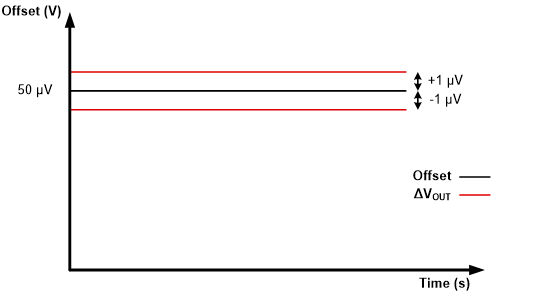##### Figure 4. Change in offset voltage due to a 100-mV change in AVDD using the ADS1261

Comparatively, wide-bandwidth applications – such as vibration monitoring – require a wider signal passband and therefore higher-bandwidth ADCs. Such ADCs are more prone to unwanted high-frequency content that may alias or fall directly into the signal bandwidth of interest. For this reason, wideband ADCs generally specify PSR using PSRRAC. Figure 5 shows the PSRR specifications for Texas Instruments’ ADS127L01, a 24-bit, high-speed delta-sigma ADC that samples up to 512 kSPS.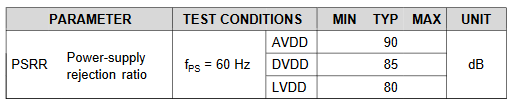##### Figure 5. ADS127L01 PSRR specifications at TA = 25°C, AVDD = 3 V, internal LVDD, DVDD = 1.8 V, VREF = 2.5 V

The measurement setup for PSRRAC is very similar to PSRRDC, such that the ADC’s inputs are shorted together and then biased to a mid-supply common-mode voltage. However, instead of introducing a DC offset to the supply, PSRRAC is measured with an AC signal riding on top of the nominal DC supply. This AC signal mimics noise at a particular frequency (for example, the 60-Hz power-line frequency shown in Figure 5).

Figure 6 shows a typical PSRRAC measurement setup with a 100-mVP sine wave on top of the ADC analog supply voltage, AVDD. If your supply voltage was 3 V, you could recreate the test setup in Figure 6 using a 100-mVPeak sine wave with a 3-V DC offset.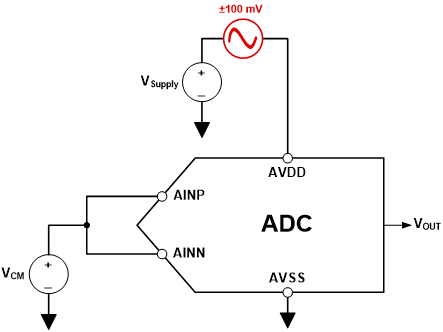##### Figure 6. PSRRAC measurement setup using a 100-mVP sine wave

To calculate PSRRAC in the time domain, you can use Equation 1 just as you did to calculate PSRRDC. Using the 100-mVP amplitude as your supply ripple (ΔVSUPPLY) and the ADS127L01’s AVDD PSRR from Figure 5 (-90 dB), you can expect that 3.2 µVP of switching ripple at 60 Hz will appear at the ADC output. Figure 7 shows the ripple on the supply and how this translates to a similar ripple at the output, centered on the ADC’s nominal offset voltage.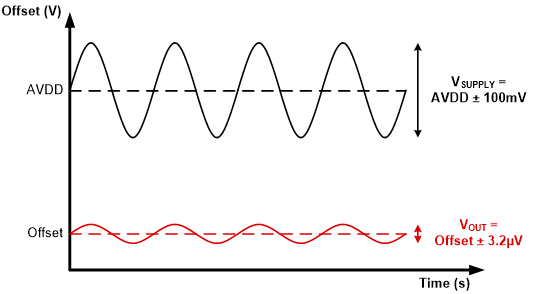##### Figure 7. Noise in ADC output due to a 100-mVP power-supply ripple using the ADS127L01

You can also calculate PSRRAC in the frequency domain by converting the 100-mVP supply ripple into decibels using the ADC’s reference voltage. If you use the 2.5-V reference voltage specified in Figure 5, 100 mVP equates to -28 dBFS, or decibels relative to full scale. The PSRRAC, in this case, is the difference in decibels between the supply-ripple amplitude and the tone measured in the frequency spectrum that appears at the supply-ripple frequency (60 Hz). Figure 8 plots both the supply-ripple amplitude and the noise that appears in the ADC output, where the difference is the direct result of the ADC’s PSRR at that frequency.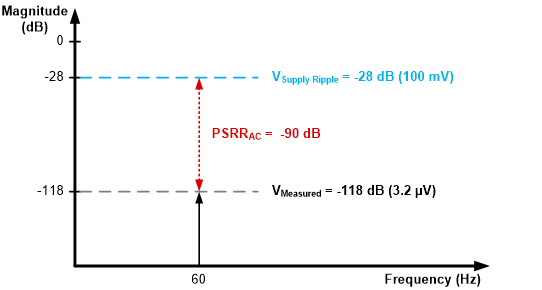### How Noise on Each of the ADCs Supplies Impacts System Performance

In the previous section, we discussed how to measure and specify power-supply noise using the analog supply as an example. While this may be acceptable for those ADCs that only require one supply voltage, higher-resolution ADCs tend to at least have separate analog and digital supplies, with some precision ADCs requiring more. For example, you can see in Figure 5 that the ADS127L01 actually has three different supplies: analog (AVDD), digital (DVDD) and the low-voltage modulator supply (LVDD). Figure 9 plots the PSRR for each supply as a function of frequency.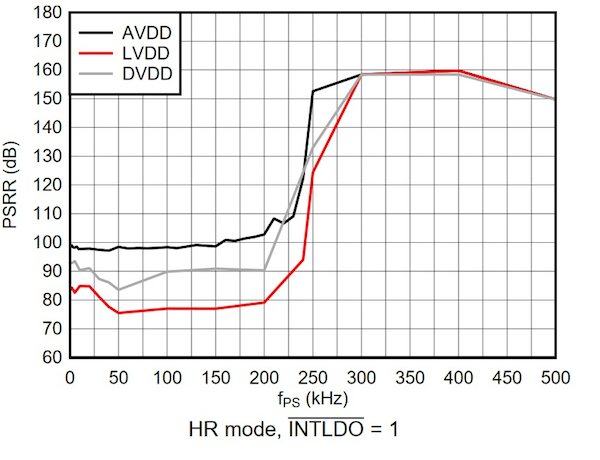##### Figure 9. TA = 25°C, AVDD = 3.3 V, VREF = 2.5 V, HR mode, ¯INTLDO = 1

You can conclude from Figure 9 that LVDD is most susceptible to power-supply noise on the ADS127L01. Intuitively, this makes sense, as this supply is directly used by the ADC’s delta-sigma modulator, which samples the input signal. For this specific ADC, then, you would want to apply some power-supply noise-reduction techniques to LVDD to ensure that it has the least amount of noise in order to maximize performance.

Additionally, you can see in Figure 9 that the PSRR for all three supplies remains relatively constant until the power-supply frequency (fPS) is approximately 200 kHz. At this frequency, the PSRR begins to increase to 160 dB for all three supplies. In Figure 9, the ADS127L01 operates at 512 kSPS using the wideband 1 digital filter. This filter response sets the ADC passband to approximately 204 kHz. Therefore, the ADS127L01’s digital filter stopband attenuation level rejects power-supply ripple at frequencies beyond the ADC passband even further – by about 116 dB. This improves the PSRR for all three supplies at higher frequencies.

These observations from Figure 9 provide hints about two important questions regarding power-supply noise: first, which ADC supply voltage is most critical when considering your system’s PSR; and second, how can you reduce the overall power-supply noise in your system?

The final article in “Resolving the Signal” will continue the discussion about power-supply noise by answering these questions with a real-world example using the ADS127L01 EVM.

Industry Articles are a form of content that allows industry partners to share useful news, messages, and technology with All About Circuits readers in a way editorial content is not well suited to. All Industry Articles are subject to strict editorial guidelines with the intention of offering readers useful news, technical expertise, or stories. The viewpoints and opinions expressed in Industry Articles are those of the partner and not necessarily those of All About Circuits or its writers.